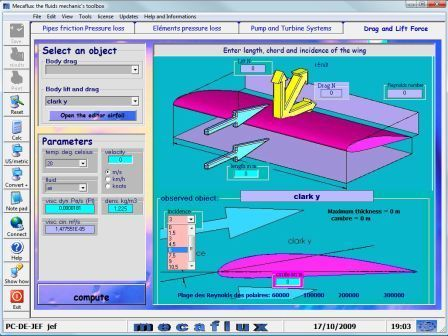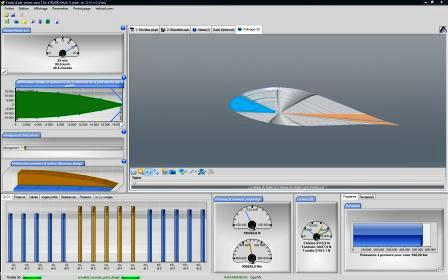We will see in this lesson a few aerodynamic concepts.

Other links further information: the propeller fan and blower , the calculation of propeller, and software propeller calculation

When an object is immersed in a fluid stream, there is phenomena of friction and turbulence.

• when the fluid is air, the study of these phenomena is the aerodynamics.

• when the fluid is water, the study of these phenomena is the Hydrodynamics .

In the air , effects of friction are studied experimentally in wind tunnel.

it is in a wind tunnel that Cd ( aerodynamics coefficient of drag) and CL (coefficient lift ) Is studied according to the Aifoils or hydrofoils .

These results are often represented as Polar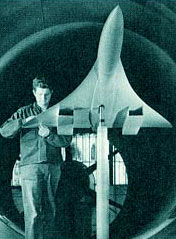concord testing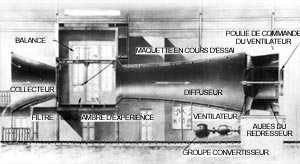wind tunnel eiffel

(the engineer who created the tower devoted the rest of his life in aeronautics research)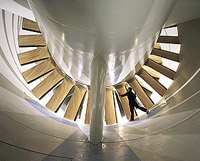NASA wind tunnel

From these experiments are derived information linking fluid characteristics and tested object.
These tests are used to calculate coefficients of lif and drag versus numbers Reynolds :The coefficient Cd drag and the aerodynamic coefficient Cl lift . The formula for determining these coefficients is that used for calculating the lift and drag. The drag and lift are known by direct measurement of forces using systems of scales or dynamometers, thus determining the Cx Cz or by simple inversion of the equation:
• Cd (drag force coefficient) p is the density fluid Kg/m3 The S front surface m² v the relative velocity of the fluid * m / s
• Drag= CD* p * s * (v²/2) become : Cx= Traînée/(p* s * (v²/2))

Drag in Newtons

• Cl (Lift force coefficient)

The Cz is the coefficient of lift, it is determined experimentally in wind tunnels or basin

p is the fluid density Kg/m3

S the projected surface ground m²

v the relative velocity of the fluid m / s

• Lift force=Cl * p * s * (v²/2) Become : Cl= Lift force/(p x s x (v²/2))
Lift force in Newtons These data are stored for years of experiments in databases. The Wright brothers had built their wind tunnel and tested their profiles. Here is an application in java retrieved from the NASA website shows their experiments. The data on the polar plate curves for different width or length ratio radius curves ... remain very useful. You can recreate your file and enter the polar data in the foil Editor available in mecaflux . the use of aerodynamic coefficient (Cd) drag and lift (Cl) is greatly facilitated in MECAFLUX because they are already entered for each selected object, you just have to choose the dimensions of your profile and parameters of fluid . If you want to use a profile that is not in the list a profiles mecaflux allows you to create one. the airfoils in the software mecaflux